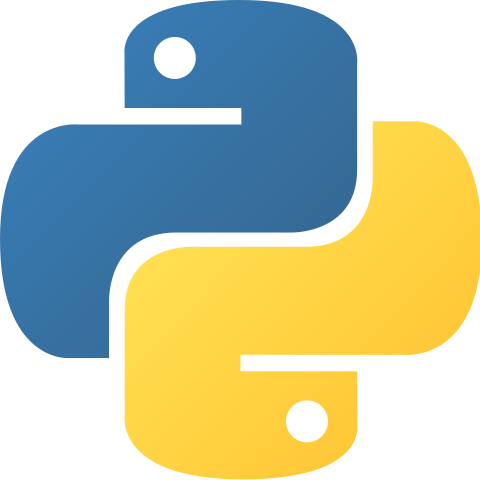# Feral Cat

##### Building, just because…## IndexError: only integers, slices (`:`), ellipsis (`…`), numpy.newaxis (`None`) and integer or boolean arrays are valid indices

Soooo you got the IndexError: only integers, slices (`:`), ellipsis (`…`), numpy.newaxis (`None`) and integer or boolean arrays are valid indices error. Let’s get that annoying message fixed.

• Python3
• macOS

#### For those Short On Time

You’re trying to access an element of a Pandas index like df.columns where df is a DataFrame by using a string most likely. i is the name of the column in the code chunk below not the row index. That was my mistake.

#### Recreating the Problem

```import pandas as pd
df = pd.DataFrame()
df['one'] = [1,2,3]
df['two'] = [1,2,3]

for i, dtype in df.dtypes.iteritems():
print(df.columns[i])
```

So the code listed above should fail. I was under the impression that i was the column number in question. Instead i is the name of the column. df.columns is an array so I’m doing [1,2,3,4][‘bleh’] which is why it’s throwing the IndexError: only integers, slices (`:`), ellipsis (`...`), numpy.newaxis (`None`) and integer or boolean arrays are valid indices error.

#### Corrected Code

```import pandas as pd
df = pd.DataFrame()
df['one'] = [1,2,3]
df['two'] = [1,2,3]

for i, dtype in df.dtypes.iteritems():
# The following line is what resolves the error and
# achieves our directive
print(i)
```

#### Lesson from all this?

So what’s the lesson from our mishap? I think I got misdirected by a few examples online that used i instead of a more descriptive variable name. Ideally that variable name would have been columnName. I always associate i with an integer which was my mistake. Hope that helps and happy coding!